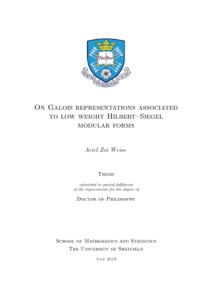# On Galois representations associated to low weight Hilbert-Siegel modular forms

Weiss, Ariel (2019) On Galois representations associated to low weight Hilbert-Siegel modular forms. PhD thesis, University of Sheffield.Preview
Text
thesis.pdf
Under the Langlands correspondence, where automorphic representations of $\GL_n$ should correspond to $n$-dimensional Galois representations, \emph{cuspidal} automorphic representations should correspond to \emph{irreducible} Galois representations. More generally, heuristically, one expects that the image of an automorphic Galois representation should be as large as possible, unless there is an automorphic reason for it to be small. This thesis addresses the consequence of this heuristic for low weight, genus $2$ Hilbert--Siegel modular forms. Let $F$ be a totally real field and $\pi=\tensor_v'\pi_v$ be a cuspidal automorphic representation of $\Gf(\AF)$, whose archimedean components lie in the holomorphic (limit of) discrete series. If $\pi$ is not CAP or endoscopic, then we show that its associated $\l$-adic Galois representation $\rho_{\pi,\l}$ is irreducible and crystalline for $100\%$ of primes. If, moreover, $\pi$ is neither an automorphic induction nor a symmetric cube lift, then we show that, for $100\%$ of primes $\l$, the image of its mod $\l$ Galois representation contains $\Sp_4(\Fl)$.